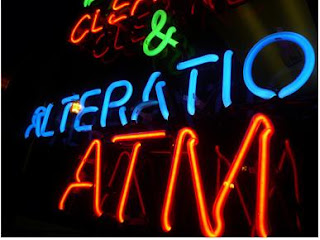## Wednesday, October 28, 2009

### Josef's BOBPart to Part Ratios:
Orange to Blue - 7:9
Vowels to Orange - 7:7
E to T - 2:3

Part to Total Ratios:
Orange to total - 7:18
Vowels to total - 7:18

Three Part Ratio:
orange to green to blue - 7:2:9

Equivalent Ratios
Equivalent Ratios are ratios that equal the same.
To make equivalent ratios you can divide or multiply, but always do what you do to one side to the other.

Ratio notation, Fraction, Decimal, and Percent

Vowels to Consonants:
V/C-7:8, = 0.875% = 87.5%

Questions:
1)what would be part to part to total ratio?
2)Show Orange to total or 7:18 ratio notation, fraction, decimal and percent
3)Make an equivalent ratio of orange to blue?